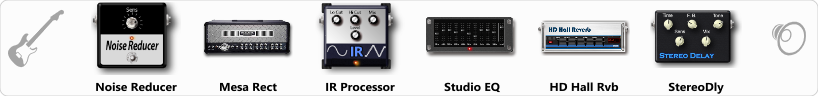# Atmospheric Black Metal Solo Tone

Discussion in 'ToneLib-GFX presets' started by Tetragramm1983, Jun 30, 2019.

1. ### Tetragramm1983Active Member

Atmospheric Black Metal Solo Tone

Preset name: Atmospheric Black Metal Rhythm 4

From Heights To Depths Solo Tone!

Effects chain:Effect: "Noise Reducer" (Dynamics / Filter), active - "yes"
"Sens" = 65
"Mode" = Hard

Effect: "Mesa Rect" (Amp simulators), active - "yes"
"Gain" = 61
"Bass" = 79
"Middle" = 20
"Treble" = 65
"Presence" = 41
"Master" = 70
"Output" = 50
"Level (dB)" = 2

Effect: "IR Processor" (Dynamics / Filter), active - "yes"
"IR" = Fortin Natas
"Low Cut (Hz)" = 128
"Hi Cut (kHz)" = 20.0
"Mix" = 100
"Level (dB)" = 6

Effect: "Studio EQ" (Dynamics / Filter), active - "yes"
"31 Hz" = 0
"62 Hz" = 0
"125 Hz" = 0
"250 Hz" = 0
"500 Hz" = 0
"1 kHz" = 3
"2 kHz" = 1
"4 kHz" = 2
"8 kHz" = 2
"16 kHz" = 0
"above 16 kHz" = 0
"Level (dB)" = 1

Effect: "HD Hall Rvb" (Reverb), active - "yes"
"Time" = 7.3
"PreLPF" = 26
"PreDelay" = 48
"HiDamp" = 38
"LoGain" = -3.3
"Mix" = 55

Effect: "StereoDly" (Delay), active - "yes"
"Time" = Note sync, 1/4
"Feedback" = 50
"Tone" = 80
"Sens" = 1
"Mix" = 70

Note: You will need to download and install the ToneLib-GFX software to use the preset.

File size:
4.9 KB
Views:
3,796